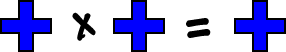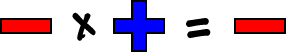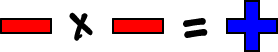Here's another one: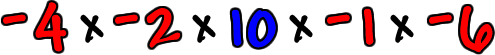If there's an even number of negatives, then the answer is positive!

(Pairs of negatives turn positive and there would be no negatives left!)

Four negatives...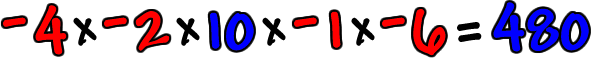(I did this one in my head by doing 2 x 4 = 8... then 8 x 6 = 48...
then 48 x 10 = 480!)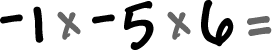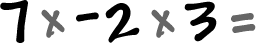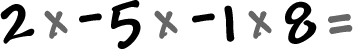So, remember these!

 The Rules: